# All About Skew Symmetric Matrix

clickHere
Updated on Oct 25, 2023 10:02 IST

A square matrix is said to be a skew-symmetric matrix if it is equal to the negative of its transpose. This article, we will briefly discuss skew-symmetric matrix, properties and theorems related to it.

A matrix is a rectangular arrangement of data points in rows and columns, and matrix with the same number of rows and columns is said to be a square matrix. This article will discuss a square matrix, which is equal to the negative of its transpose, i.e., a skew-symmetric matrix.

In the previous article, we discussed the symmetric matrix; the properties and theorems of the skew-symmetric matrix will be very similar to the symmetric matrix.

Before starting the article, let’s discuss some of the properties of the transpose of a matrix.

• (A+B)T = AT + BT
• (kA)T = kAT, where k is some scalar
• (AT)-1 = (A-1)T, where A is an invertible matrix
• (AT)T = A
• (AB)T = BTAT

We will use these properties in the chapter while proving the theorems.

Must Check: Top Math Courses for Data Science

Now, let’s explore Skew-Symmetric Matrix, its definition, properties, and theorems.

## What is a Skew-Symmetric Matrix?

### Definition

A square matrix that is equal to the negative of its transpose is called a skew-symmetric matrix, i.e., a square matrix is said to be a skew-symmetric matrix if and only if:

A = -AT

### Representation

If B = [bij]n, is a skew-symmetric matrix, if bij = -bji, for all

• 1<= i, j <= n, for all n belongs to Natural Number.
• bij represents the element at the i th row and j th column.Difference between Eigenvalue and Eigenvector
Let A be a square matrix of order ‘n-by-n.’ A scalar k is called a eigenvalue of A, if there exist a non-zero vector v satisfying Av = kv, then...read more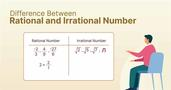Difference Between Rational and Irrational Number
Numbers are an integral part of our lives that are used to quantify, measure, and calculate everything from the amount of time we spend on our smartphones to the distance...read moreDifference between Permutation and Combination
In mathematics, Permutation and Combination are one of the most confusing topics as they are closely related. Permutation counts the number of different arrangements from n objects; on the other...read more

### Example: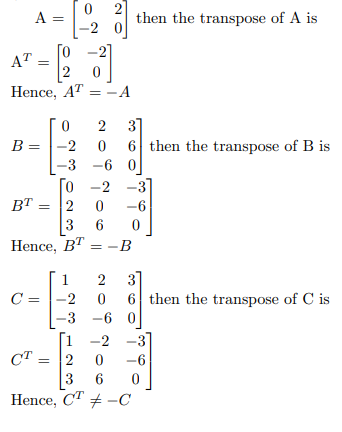Here, we have taken three examples: first two examples are skew-symmetric in nature, since

AT = -A, and B T = -B.

But, in example – 3, C T != -C

Hence, C is not a skew-symmetric matrix.

Must Check: Free Maths for Data Science Online Courses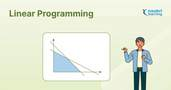Linear Programming Problem (LPP)
Linear Programming problem or LPP is a method to find the optimum solution of set of parameters that are represented in linear form. In this article, we will discuss all...read moreTransportation Problem: Definition, Formulation, and Types
Transportation problems are used to find the minimum cost of transportation of goods from m source to n destination. In this article we will learn transportation problem, formulation, types and...read more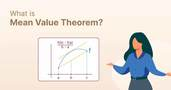The Mean Value Theorem in Data Science: A Comprehensive Guide
Mean value theorem or Lagrange mean value theorem states that if f(x) is a continuous and differentiable function over an interval (a, b), then there exists at least one c...read more

## Properties

• The Sum of two skew-symmetric matrices is always a skew-symmetric matrix.

(A + B)T = A T + B T = -A – B = – (A + B)

Hence, A + B = -(A + B) T

• The scalar multiplication of a skew-symmetric matrix is always skew-symmetric.

(kA)T = k(A)T = k(-A) = -kA

Hence, (kA)T = -kA

• If A and B are skew-symmetric matrices, such that AB = -BA, then AB is a skew-symmetric matrix.

(AB) T = B T A T = (-B)(-A) = BA = -AB

Hence, (AB) T = -AB

• The diagonal elements of a skew-symmetric matrix is always zero.

Let A be a skew-symmetric matrix, then

aij = -aji

Now, for the diagonal entries, the above will be:

aii = -aii

aii + aii = 0

2aii = 0

aii = 0

• The Trace (i.e., the sum of all elements of the principal diagonal) of a skew-symmetric matrix is always zero.
• The Sum of a skew-symmetric matrix and the identity matrix is always an invertible matrix.
• The inverse of an invertible skew-symmetric matrix is always a skew-symmetric matrix.

Let A be a skew-symmetric matrix:

A = -A T

Taking inverse both the side, we get:

A-1 = (-A T ) -1 = -(A-1) T

Hence,

A-1 = -(A-1) T

• Let v be an n-dimensional column vector, then vTAv = 0, where A is a skew-symmetric matrix.
• Determinant of a skew-symmetric matrix
• The eigenvalues of skew-symmetric are always zero or imaginary.

Check our Web Story: Top 10 Most Wanted Data Science SkillsScalars and Vectors: Understanding the Key Differences
Physical quantity with magnitude and no direction is known as a scalar quantity and a physical quantity with magnitude and directions is known as a vector quantity. In this article,...read moreHow Vectors are Used in Machine Learning
Vectors are mathematical objects that contain both magnitude and direction, and they can be represented by the directed line segments (lines having directions) whose lengths are their magnitude. It is...read moreCross Product of two Vectors
Cross product is a binary operation (multiplication) that is performed on two vectors, and the resultant vector is perpendicular to both the given vectors. In this article, we will discuss...read more

## Theorem

### Theorem – 1: For any real square matrix A, A – AT will be a skew-symmetric matrix.

Given: A is a real square matrix.

To Prove: A – AT is a skew-symmetric matrix.

Concepts to be used:

• (A+B)T = AT + BT
• (kA)T = kAT, where k is some scalar
• (AT)T = A

Proof:

Let B be a skew-symmetric matrix such that

B = A – AT

Now, taking the transpose both the side, we get

B T = (A – A T ) T = A T + (-A T ) T = A T – A = – (A – A T ) = -B

Hence, A – A^T is a skew-symmetric matrix.

### Theorem – 2: Every Square matrix can be decomposed uniquely as a sum of the symmetric and skew-symmetric matrices.

i.e., for any square matrix A,

A = (½) [S +V], where

S = A + A T , and V = A – A T

To know the proof of the above theorem, check out: All about Symmetric Matrix.

### Theorem – 3: If A is a skew-symmetric matrix, then BTAB is a skew-symmetric matrix.

Given: A is a skew-symmetric matrix, and B is any matrix.

To Prove: B T AB is a skew-symmetric

Concepts to be used:

• (kA) = kAT, where k is some scalar
• (AT)T = A
• (AB)T = BTAT

Proof:

(BTAB) T = [ B T (AB)] T = (AB) T (B T ) T = B T A T B = B T (-A)B = -B T AB

Hence,

(B T AB) T = – -B T AB

Thus, if A is a skew-symmetric matrix, then B T AB is a skew-symmetric matrix.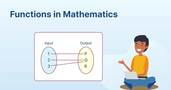Function in Mathematics: Definition, Types, and Examples
A relation from a non-empty set A to a non-empty set B is said to be a function from A to B if and only if every element of the...read moreUnderstanding Set Theory – What, Where, Why, and How do we use it in Data Science
Set in mathematics is a well-defined collection of objects that doesn’t vary from person to person. In this article, we will briefly discuss set theory, its representation, subset, cardinality, union...read more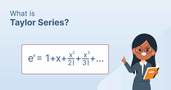Taylor Series Approximation: Definition, Formula, and Example
Let f(x) be a continuous and infinitely differentiable function (i.e., a function that can be differentiated infinite times), then the taylor series of f(x) is a series expansion of f(x) about a point x = a. In...read more

## Conclusion

In this article, we have briefly discussed skew-symmetric matrix, their properties and theorems related to it.

Hope you will like the article.

Keep Learning!!
Keep Sharing!!

Must Check: Mathematics for Machine Learning

## FAQs

What is Skew-Symmetric Matrix?

A square matrix that is equal to the negative of its transpose is called a skew-symmetric matrix

What are the different properties of Skew-Symmetric Matrix?

1. The Sum of two skew-symmetric matrices is always a skew-symmetric matrix. 2. The scalar multiplication of a skew-symmetric matrix is always skew-symmetric. 3. If A and B are skew-symmetric matrices, such that AB = -BA, then AB is a skew-symmetric matrix. 4. The diagonal elements of a skew-symmetric matrix is always zero. 5. The Sum of a skew-symmetric matrix and the identity matrix is always an invertible matrix.

How do you determine which matrix is a skew-symmetric matrix?

To check if a given matrix is a skew-symmetric matrix or not, you have to check two conditions only: 1. Given matrix must have been a square matrix. 2. Transpose of a matrix is equal to its negative times of the matrix, i.e., (A^T) = -A.

What is the eigen value of the skew-symmetric matrix?

The eigen value of skew symmetric matrix is either zero or purely imaginary.

Can a skew symmetric matrix be diagonalized?

Yes, a skew symmetric matrix can be diagonalized, particularly over the complex field. The process involves finding a basis consisting of eigenvectors and then transforming the matrix into a diagonal form using these eigenvectors. The diagonal elements will be the eigenvalues, which are either zero or purely imaginary for real skew symmetric matrices.

How is a skew symmetric matrix used in real-world applications?

Skew symmetric matrices are widely used in areas such as physics, particularly in the study of angular momentum, and in the representation of cross products in vector spaces. They also appear in mathematical areas like differential geometry and the theory of Lie groups and Lie algebras.

Are skew symmetric matrices invertible?

Skew symmetric matrices can be invertible, but not always. A skew symmetric matrix is invertible if and only if its determinant is non-zero. However, for even order skew symmetric matrices, the determinant is always zero, making them non-invertible. For odd order, it depends on the specific elements of the matrix.

clickHere

Vikram has a Postgraduate degree in Applied Mathematics, with a keen interest in Data Science and Machine Learning. He has experience of 2+ years in content creation in Mathematics, Statistics, Data Science, and Mac... Read Full Bio

## Trending Data Science CoursesData Science with Python
UDEMY4.2AI For Everyone
Coursera4.5Become a Data Science Professional with IIT Certification in Advanced ProgrammingBecome a Data Science Professional with IIT Certif...
Guvi5.0Become a Data Science Professional with IIT Certification in Advanced ProgrammingBecome a Data Science Professional with IIT Certif...
Guvi5.0Starts 15 Dec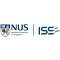## Top Picks & New Arrivals